# Introduction to bridging Olink® NPX datasets

#### Compiled: November 03, 2023

Individual Olink® NPX datasets are normalized using either plate control normalization or intensity normalization methods. Plate control normalization is generally used for single plate projects or for Explore HT projects, while intensity normalization is generally used for multiplate projects. Additionally, intensity normalization method assumes that all samples within a project are fully randomized.

In the case where all samples within a project are not fully randomized, or when a study is separated into separate batches, an additional normalization step is needed to allow the data to be comparable, since NPX is a relative measurement. The joint analysis of two or more Olink® NPX datasets often requires an additional batch correction step to remove technical variations, which is referred to as overlapping sample reference normalization, bridge normalization, or just simply bridging.

Bridging is also needed if Olink® NPX datasets are:

• plate control normalized only and run conditions (e.g lab and reagent lots) have changes.

• intensity normalized but from two different sample populations.

To bridge two or more Olink® NPX datasets, bridging samples are needed to calculate the assay-specific adjustment factors between datasets. Bridging samples are shared samples among datasets - that is that samples that are analyzed in both datasets. The recommended number of bridging samples are shown in the table below. Olink® NPX datasets without shared samples should not be combined using the bridging approach described below.

Table 1. Recommended number of bridging samples for Olink platforms
Platform BridgingSamples
Target 96 8-16
Explore 384 Cardiometabolic, Inflammation, Neurology, and Oncology 8-16
Explore 384 Cardiometabolic II, Inflammation II, Neurology II, and Oncology II 16-24

The following tutorial is designed to give you an overview of the kinds of data combining methods that are possible using the Olink® bridging procedure. Before starting bridging, it is important to check if the same sample IDs were assigned to the bridging samples.

## Selecting bridging samples

Prior to running the second study, bridging samples must be selected from the reference study and be added to the second study. These samples can be selected using the olink_bridgeselector() function in Olink Analyze. The bridge selection function will select a number of bridge samples based on the reference data. This function selects samples which passes QC and have high detectibility. In the case of where detectibility cannot be calculated from the test data set (ex: Explore HT data), the function will only select samples which pass QC. External controls are not selected as bridge samples as they are not necessarily representative of the study and therefore may not cover the dynamic range of assays that would be expressed within the samples.

To select samples across the range of the data, the samples are ordered by mean NPX value and selected across this range. When running the selector, Olink recommends starting at sampleMissingFreq = 0.10 which represents a maximum of 10% data below LOD per sample. If there are not enough samples output, increase to 20%. For alternative matrices and specific disease types, it may be needed to increase the sampleMissingFreq to higher levels.

In this example we will demonstrate how to select 16 bridging samples using npx_data1 which will act as the reference data. The selected bridge samples are displayed in Table 2.

bridge_Samples<-olink_bridgeselector(df = npx_data1,
sampleMissingFreq = 0.1,
n = 16)
Table 2. Selected Bridging Samples
SampleID PercAssaysBelowLOD MeanNPX
A67 0.04 6.35
A20 0.05 6.54
A47 0.07 6.15
A53 0.04 6.28
B20 0.06 6.46
B13 0.05 6.32
B9 0.06 5.99
B55 0.08 6.26
B35 0.07 6.24
A77 0.07 6.22
B65 0.05 6.39
B36 0.07 6.20
B79 0.06 6.11
A59 0.03 6.07
B62 0.05 6.08
B34 0.05 5.87

It’s important to make sure that the select bridge samples are representative of the overall samples within the study. This can be done by generating a PCA plot with the following code and make sure that the bridge samples are evenly dispersed among the other samples, as shown in Figure 1.

npx_data1 %>%
filter(!str_detect(SampleID, 'CONT')) %>%
mutate(Bridge = ifelse(SampleID %in% bridge_Samples$SampleID, "Bridge", "Sample")) %>% olink_pca_plot(color_g = "Bridge")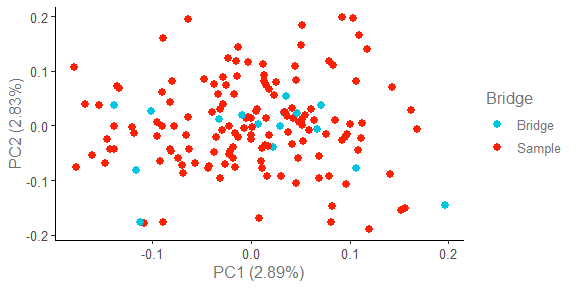Figure 1. PCA plot of bridging samples and other samples in npx_data1. Control samples are excluded from the PCA plot. ## Setup bridging datasets Bridging datasets are standard Olink® NPX tables. They can be loaded using read_NPX() function with default Olink Software NPX file as input. data1 <- read_NPX("~/NPX_file1_location.xlsx") data2 <- read_NPX("~/NPX_file2_location.xlsx") To demonstrate how bridging works, we will use the example datasets (npx_data1 and npx_data2) from Olink Analyze package. This workflow also uses functions from the dplyr, stringr, and ggplot2 packages. ## Check bridging datasets First, confirm that there are overlapping sample IDs within the study. It is important that the sample IDs are the same in both NPX files as shown in Table 3. Note that while external controls may share the same SampleID in both datasets, theses samples should not be treated as bridging samples. External control samples often share the same naming convention across datasets but may represent different samples due to reagent batch differences. Additionally, external control samples are unlikely to cover the dynamic range of assays expressed in the study samples. data.frame(SampleID = intersect(npx_data1$SampleID, npx_data2$SampleID)) %>% dplyr::filter(!stringr::str_detect(SampleID, "CONTROL_SAMPLE")) Table 3. Overlapping Bridge Samples SampleID A13 A29 A30 A36 A45 A46 A52 A63 A71 A73 B3 B4 B37 B45 B63 B75 Then, gain an overview of the datasets that are going to be bridged. For example, plot and compare NPX distribution between datasets, as shown in Figure 2. By having a sense of how the studies compared to each other before bridging, we can then determine the success of the bridging process post bridging. Figure 2 shows a large overlap between data1 and data2, however there are still some differences as indicated by the blue and red areas on the edge of the distribution. # Load datasets npx_1 <- npx_data1 %>% mutate(Project = "data1") npx_2 <- npx_data2 %>% mutate(Project = "data2") npx_df <- bind_rows(npx_1, npx_2) # Plot NPX density before bridging normalization npx_df %>% mutate(Panel = gsub("Olink ", "", Panel)) %>% ggplot(aes(x = NPX, fill = Project)) + geom_density(alpha = 0.4) + facet_grid(~Panel) + olink_fill_discrete(coloroption = c("red", "darkblue")) + set_plot_theme() + ggtitle("Before bridging normalization: NPX distribution") + theme(axis.title.x = element_blank(), axis.title.y = element_blank(), strip.text = element_text(size = 16), legend.title = element_blank(), legend.position = "top")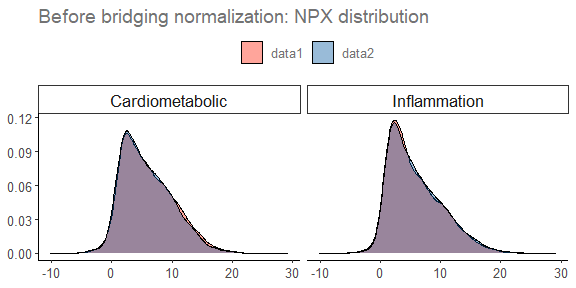Figure 2. Density plot of NPX distribution in both datasets before bridging. Use a PCA plot as shown in Figure 3 to visualize sample-to-sample distance before bridging. Typically the project dataset accounts for most of the observed variation within the combined datasets at this point. Control samples are removed from the PCA plot because they are not expected to be representative of the rest of the dataset, and therefore the variation between sample and control samples could skew the PCA, making it more challenging to see differences between projects. Additional, the PCA function does not support multiple samples with the same SampleID, which is also why the bridging samples in the second project are appended with “_new”. In Figure 3, data1 and data2 separate along PC1, indicating batch differences between the two datasets. #### Extract bridging samples overlapping_samples <- data.frame(SampleID = intersect(npx_1$SampleID, npx_2$SampleID)) %>% filter(!str_detect(SampleID, "CONTROL_SAMPLE")) %>% #Remove control samples pull(SampleID) npx_before_br <- npx_data1 %>% dplyr::filter(!str_detect(SampleID, "CONTROL_SAMPLE")) %>% #Remove control samples dplyr::mutate(Type = if_else(SampleID %in% overlapping_samples, paste0("data1 Bridge"), paste0("data1 Sample"))) %>% rbind({ npx_data2 %>% filter(!str_detect(SampleID, "CONTROL_SAMPLE")) %>% #Remove control samples %>% mutate(Type = if_else(SampleID %in% overlapping_samples, paste0("data2 Bridge"), paste0("data2 Sample"))) %>% mutate(SampleID = if_else(SampleID %in% overlapping_samples, paste0(SampleID, "_new"), SampleID)) }) ### PCA plot OlinkAnalyze::olink_pca_plot(df = npx_before_br, color_g = "Type", byPanel = TRUE)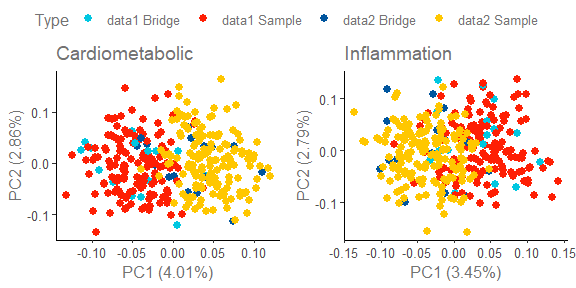Figure 3. PCA plot of both datasets before bridging. ## Perform bridging between two data sets We can use olink_normalization_bridge() function to bridge two datasets. The bridging procedure is to first calculate the median of the paired NPX differences per assay between the bridging samples as adjustment factor then use these adjustment factors to adjust NPX values between two datasets. In this process, one dataset is considered the reference dataset (df1) and its NPX values remain unaltered. The other dataset is considered the new dataset (df2) and is adjusted to the reference dataset based on the adjustment factors. The output from olink_normalization_bridge() function is a NPX table with adjusted NPX value in the column NPX, as shown in Table 4. olink_normalization_bridge() is a wrapper for and supersedes olink_normalization() . olink_normalization_bridge() creates a new column Project to distinguish between reference dataset from the other dataset. It is up to the user to define which dataset is the reference dataset and specify the names of the bridge samples. The resulting dataset will contain the reference dataset, which will be identical to the input reference data, with adjustment factors of 0, and the newly bridged dataset. # Find shared samples npx_1 <- npx_data1 %>% mutate(Project = "data1") npx_2 <- npx_data2 %>% mutate(Project = "data2") overlap_samples <-data.frame(SampleID = intersect(npx_1$SampleID, npx_2\$SampleID)) %>%
filter(!str_detect(SampleID, "CONTROL_SAMPLE")) %>% #Remove control samples
pull(SampleID)

overlap_samples_list <- list("DF1" = overlap_samples,
"DF2" = overlap_samples)

# Perform Bridging normalization
project_2_df = npx_2,
bridge_samples = overlap_samples_list,
project_1_name = "data1",
project_2_name = "data2",
project_ref_name = "data1")
Table 4. First 10 rows of combined datasets after bridging.
Index OlinkID UniProt Assay MissingFreq Panel_Version PlateID QC_Warning LOD NPX Subject Treatment Site Time Project Panel Adj_factor SampleID
1 OID01216 O00533 CHL1 0 v.1201 Example_Data_1_CAM.csv Pass 2.4 13.0 ID1 Untreated Site_D Baseline data1 Olink Cardiometabolic 0 A1
2 OID01216 O00533 CHL1 0 v.1201 Example_Data_1_CAM.csv Pass 2.4 11.3 ID1 Untreated Site_D Week.6 data1 Olink Cardiometabolic 0 A2
3 OID01216 O00533 CHL1 0 v.1201 Example_Data_1_CAM.csv Pass 2.4 25.5 ID1 Untreated Site_D Week.12 data1 Olink Cardiometabolic 0 A3
4 OID01216 O00533 CHL1 0 v.1201 Example_Data_1_CAM.csv Pass 2.4 14.5 ID2 Untreated Site_C Baseline data1 Olink Cardiometabolic 0 A4
5 OID01216 O00533 CHL1 0 v.1201 Example_Data_1_CAM.csv Pass 2.4 7.6 ID2 Untreated Site_C Week.6 data1 Olink Cardiometabolic 0 A5
6 OID01216 O00533 CHL1 0 v.1201 Example_Data_1_CAM.csv Pass 2.4 6.3 ID2 Untreated Site_C Week.12 data1 Olink Cardiometabolic 0 A6
7 OID01216 O00533 CHL1 0 v.1201 Example_Data_1_CAM.csv Pass 2.4 12.7 ID3 Untreated Site_D Baseline data1 Olink Cardiometabolic 0 A7
8 OID01216 O00533 CHL1 0 v.1201 Example_Data_1_CAM.csv Pass 2.4 13.7 ID3 Untreated Site_D Week.6 data1 Olink Cardiometabolic 0 A8
9 OID01216 O00533 CHL1 0 v.1201 Example_Data_1_CAM.csv Pass 2.4 16.2 NA NA NA NA data1 Olink Cardiometabolic 0 CONTROL_SAMPLE_AS 1
10 OID01216 O00533 CHL1 0 v.1201 Example_Data_1_CAM.csv Pass 2.4 6.1 ID3 Untreated Site_D Week.12 data1 Olink Cardiometabolic 0 A9

## Perform bridging with non-matching sample names

olink_normalization_bridge() also supports data where the shared samples (bridge samples) are not named the same in both projects. In this case the overlap_sample_list will contain 2 arrays of equal length where the index of each entry corresponds to the same sample. For example, if a sample had the SampleID of Sample_1_Aliquot_1 in the first batch and Sample_1_Aliquot_2 in the second batch, then the overlap sample list should look as the following.

overlap_sample_list <-list("DF1" = c("A1", "A2", "A3", "Sample_1_Aliquot_1"),
"DF2" = c("A1", "A2", "A3", "Sample_1_Aliquot_2")) 

## Perform evaluation of bridging normalization

First, check NPX distribution in datasets after bridging normalization, as shown in Figure 4. Figure 4 shows a larger overlap in projects than seen in Figure 2, as indicated by fewer areas of red and blue along the outside of the distribution.

# Plot NPX density after bridging normalization

npx_br_data %>%
mutate(Panel = gsub("Olink ", "", Panel)) %>%
ggplot2::ggplot(ggplot2::aes(x = NPX, fill = Project)) +
ggplot2::geom_density(alpha = 0.4) +
ggplot2::facet_grid(~Panel) +
set_plot_theme() +
ggplot2::ggtitle("After bridging normalization: NPX distribution") +
theme(axis.title.x = element_blank(),
axis.title.y = element_blank(),
strip.text = element_text(size = 16),
legend.title = element_blank(),
legend.position = "top")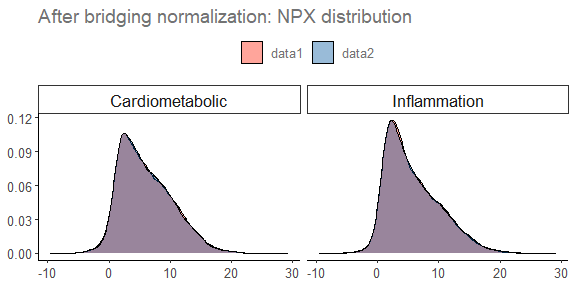Figure 4. Density plot of NPX distribution in both datasets after bridging.

Then, summarize number of assays that have adjustment factors in certain ranges. High adjustment factors can result from variations between projects, such as panel versions or technical modifications. The cutoff of a deviating adjustment factor is subjective and depends on a variety of factors including the distribution of adjustment factors, as shown in Figure 5. While there are a few assays with adjustment factors between 2 and 4 and -2 and -4, the majority of the adjustment factors shown in Figure 5 occur between -2 and 2.

Such assays can be visualized individually with violin plots, as shown in Figure 6, and may warrant further investigation to confirm they are still comparable between projects. For example, if a violin plot exhibits a different range or truncated distribution, this may suggest that the assay is below LOD or at hook in one of the data sets. However, as long as the bridge samples are not at hook or below LOD, this should not impact the bridging quality. For projects with differing clinical phenotypes, it is more informative to look at the similarities between the bridging samples than the similarity between the datasets, as indicated by the overlaid black dots in Figure 6.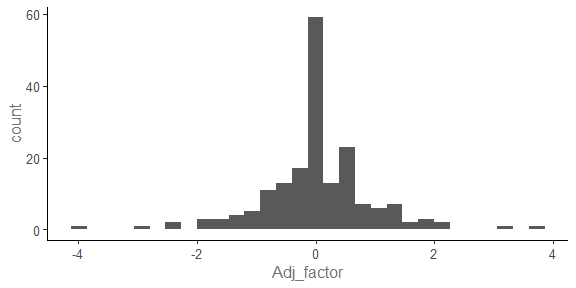Figure 5. Histogram of adjustment factors in normalized data from Project “data2”.

The distribution of CHL1 between projects is visualized for demonstration purposes.

# Bridge sample data
bridge_samples <- npx_1 %>%
rbind(npx_2) %>%
filter(SampleID %in% overlapping_samples) %>%
filter(Assay == "CHL1") %>%
mutate(Assay_OID = paste(Assay, OlinkID, sep = "\n"))

# Generate violin plot for CHL1
npx_data1 %>%
mutate(Project = "data1") %>%
bind_rows({
npx_data2 %>%
mutate(Project = "data2")
}) %>%
filter(Assay == "CHL1") %>%
filter(!str_detect(SampleID, "CONTROL*.")) %>%
mutate(Assay_OID = paste(Assay, OlinkID, sep = "\n")) %>%
ggplot2::ggplot(aes(Project, NPX)) +
ggplot2::geom_violin(aes(fill = Project)) +
geom_point(data = bridge_samples, position = position_jitter(0.1)) +
theme(legend.position = "none") +
set_plot_theme() +
facet_wrap(. ~ Assay_OID, scales='free_y')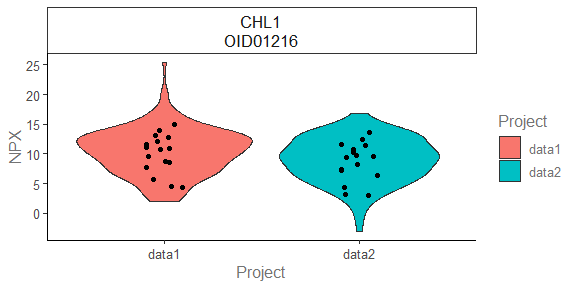Figure 6. Violin plot of CHL1 in both datasets prior to bridging. Bridge samples are indicated by black points.

Another way to determine if bridging decreased variability between projects is to calculate the CV of the control samples across both projects before and after bridging, as shown in Figure 7. The CV after normalization is expected to be smaller than the CV prior to normalization, as shown in Figure 7.

Note that the CV calculation formula differs for Target 96 and Explore projects.


explore_cv <- function(npx, na.rm = F) {
sqrt(exp((log(2) * sd(npx, na.rm = na.rm))^2) - 1)*100
}

t96_cv <- function(NPX, na.rm = T) {
100*sd(2^NPX)/mean(2^NPX)
}

tech <- "Target"

cv_before <- npx_1 %>%
rbind(npx_2) %>%
filter(str_detect(SampleID,"CONTROL*.")) %>%
filter(NPX > LOD) %>%
mutate(CV = ifelse(tech=='Explore',explore_cv(NPX), t96_cv(NPX))) %>%
ungroup() %>%

cv_after <- npx_br_data %>%
filter(str_detect(SampleID, "CONTROL")) %>%
filter(NPX > LOD) %>%
mutate(CV = ifelse(tech=='Explore',explore_cv(NPX), t96_cv(NPX))) %>%
ungroup() %>%

cv_before %>%
mutate(Analysis = "Before") %>%
rbind((cv_after %>%
mutate(Analysis = "After"))) %>%
ggplot2::ggplot(ggplot2::aes(x = CV, fill = Analysis)) +
ggplot2::geom_density(alpha = 0.7) +
set_plot_theme() +
ggplot2::theme(text = ggplot2::element_text(size = 20)) + ggplot2::xlim(-50,400)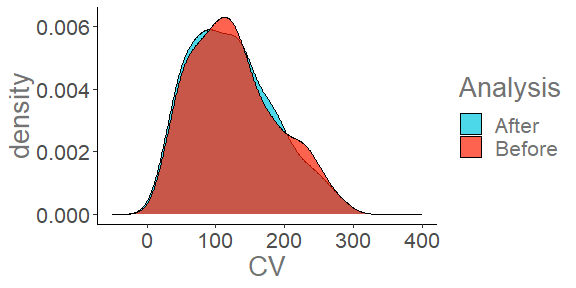Figure 7. Density plot of inter-project CV before and after bridging.

Finally, use PCA plot to check whether bridging normalization has effect in correcting batch effects (Figure 8). In the example below, it is clear that before bridging samples from data 1 and 2 are divided into separate clusters due to the batch effects (Figure 3), but after bridging they are shown as one cluster in the PCA plot (Figure 8). Bridging normalization has sufficiently removed the batch effects between two data sets.

## After bridging

### Generate unique SampleIDs

npx_after_br <- npx_br_data %>%
dplyr::mutate(Type = ifelse(SampleID %in% overlapping_samples,
paste(Project, "Bridge"),
paste(Project, "Sample"))) %>%
dplyr:::mutate(SampleID = paste0(Project, PlateID, SampleID))

### PCA plot
color_g     = "Type",
byPanel     = TRUE)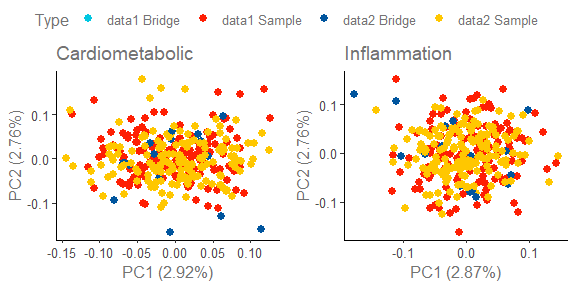Figure 8. PCA plot of both datasets after bridging.

## Export Bridged data

Normalized data can be exported using write.table to export long format data. Note that 2 columns are added during the bridging process, so to have the input format match the export format the Project and Adj_factor columns will need to be removed. To export the new project, dplyr::filter can be used to filter by Project.

new_normalized_data <- npx_br_data %>%
dplyr::filter(Project == "data2") %>%
write.table(, file = "New_Normalized_NPX_data.csv", sep = ";")